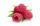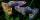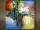# Cuboid

The sum of the lengths of the three edges of the cuboid that originate from one vertex is 210 cm. Edge length ratio is 7: 5: 3. Calculate the length of the edges.

Result

a =  98 cm
b =  70 cm
c =  42 cm

#### Solution:Leave us a comment of example and its solution (i.e. if it is still somewhat unclear...):Be the first to comment!## Next similar examples:

1. Simplify 2Simplify expression: 5ab-7+3ba-9
2. RaspberriesDano had 20 raspberries. John had 90% more raspberries than Dano. Determine how many raspberries have Dano and Juraj together.
3. Unknown number 24f we add 20, we get 50% of its triple. What is this unknown number?
4. GuppiesAudrey has some guppies in a fish tank. The ration of the oranges guppies to silver guppies is 3:5. She has 12y oranges guppies. Write the number of silver guppies she has in terms of y
5. Greg and BillGreg is 18 years old. He is 6 less than 4 times Bill's age. How old is Bill?
6. Simple equationsSolve system of equations: 5x+3y=5 5x+7y=25
7. Two machinesPerformances of two machines are in a ratio of 7:12. A machine with less power produced 406 pieces of products per shift. a) How many pieces produced per shift second machine? b) How many pieces produced two machines together for five shifts?
8. DonutsFind how many donuts each student will receive if you share 126 donuts in a ratio of 1:5:8Combine like terms 4c+c-7c
10. Image scaleThe actual image dimensions are 60 cm x 80cm and has a reduced size 3 cm x 4 cm. At what scale the image was reduced?
11. PeppersIn the box are yellow (a), green (b) and red (c) peppers. Their amount is in a ratio 2:4:1 . Most are yellow peppers and green the least. Calculate the number of peppers each type if the total number of peppers is 70.
12. AgesJohn, Teresa, Daniel and Paul have summary 56 years. Their ages are in a ratio of 1:2:5:6. Determine how many years have each of them.
13. Land areaA land area of Asia and Africa are in a 3: 2 ratio, the European and African are is 1:3. What are the proportions of Asia, Africa, and Europe?
14. Line segmentThe 4 cm long line segment is enlarged in the ratio of 5/2. How many centimeters will measure the new line segment?
15. Two numbersFind two numbers whose difference and ratio is 2.
16. Arble bagA marble bag sold by Rachel's Marble Company contains 5 orange marbles for every 6 green marbles. If a bag has 35 orange marbles, how many green marbles does it contain?
17. 1.5 divided1.5 divided by 1 = w divided by 4